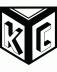## Bouncy balls with Perl 6

I've never written games before, but I previously posted a Hangman that I thought was fun. I love the examples of forest fire and Game of Life and wanted to create something like those. I originally wanted to create Pong but decided to try a simple ball that bounces off the walls of a container.

I showed this to my daughter, and she asked if there could be more than one ball, so I added that. They don't, however, bounce off each other, and this gets at the same problem I was mulling with regard to adding obstacles in the field that should deflect the balls. I have a feeling that two-dimensional arrays would help me add this feature. Support for that is coming. Anyway, on to the script!

```#!/usr/bin/env perl6

my enum HorzDir <Left Right>;
my enum VertDir <Up Down>;

subset PosInt of Int where * > 0;

class Ball {
has Int \$.rows;
has Int \$.cols;
has Int \$.row is rw = (1..\$!rows).pick;
has Int \$.col is rw = (1..\$!cols).pick;
has HorzDir \$.horz-dir is rw = HorzDir.pick;
has VertDir \$.vert-dir is rw = VertDir.pick;

method Str { "(\$.row, \$.col)" }

method reverse-horz-dir {
\$!horz-dir = \$!horz-dir == Left ?? Right !! Left;
}

method reverse-vert-dir {
\$!vert-dir = \$!vert-dir == Down ?? Up !! Down;
}

method move {
if \$!horz-dir == Right {
\$!col += \$!col < \$!cols ?? 1 !! -1;
}
else {
\$!col += \$!col > 1      ?? -1 !! 1;
}

if \$!vert-dir == Down {
\$!row += \$!row < \$.rows ?? 1 !! -1;
}
else {
\$!row += \$!row > 1      ?? -1 !! 1;
}

\$.reverse-horz-dir if \$!col <= 1 || \$!col >= \$.cols;
\$.reverse-vert-dir if \$!row <= 1 || \$!row >= \$.rows;
}
}

my \$DOT           = "\x25A0"; # ■
my \$STAR          = "\x2605"; # ★
my \$SMILEY-FACE   = "\x263A"; # ☺
my (\$ROWS, \$COLS) = qx/stty size/.words;

sub MAIN (
PosInt :\$rows=\$ROWS - 4,
PosInt :\$cols=\$COLS - 2,
PosInt :\$balls=1,
Numeric :\$refresh=.1,
Bool :\$smiley=False,
Bool :\$star=False,
) {
print "\e[2J";
my Str \$bar    = '+' ~ '-' x \$cols ~ '+';
my \$icon       = \$smiley ?? \$SMILEY-FACE !! \$star ?? \$STAR !! \$DOT;
my Ball @balls = do for ^\$balls { Ball.new(:\$rows, :\$cols) };

loop {
.move for @balls;

print "\e[H";
my \$screen = "\$bar\n";

for 1..\$rows -> \$this-row {
my \$line = '|' ~ " " x \$cols;

for @balls -> \$ball {
if \$this-row == \$ball.row {
\$line.substr-rw(\$ball.col, 1) = \$icon;
}
}

\$screen ~= "\$line|\n";
}

\$screen ~= \$bar;
put \$screen;
sleep \$refresh;
}
}
```

There is a ton packed into this code, mostly because Perl's objects have such a compact syntax. There are so many new features I love in the language such as "subset" to create custom types and "enum" to create type-ish things. I can't imagine doing without my "MAIN" anymore, esp with the way it turns the amazing subroutine signatures into usage statements.

Thanks to the #perl6 IRC channel for suggestion on improving my code and answering all my questions.

Source in Github. Also, read my book.

UPDATE
Thanks to suggestions from Reddit, I herewith submit a new version with one very big improvement -- the balls fight to the death now! I agree that the enum stuff was a bit belabored. The suggestions for the "move" method were much better than what I had.

```#!/usr/bin/env perl6

subset PosInt of Int where * > 0;

class Ball {
has Int \$.rows;
has Int \$.cols;
has Int \$.row is rw = (2..^\$!rows).pick;
has Int \$.col is rw = (2..^\$!cols).pick;
has Int \$.horz-dir is rw = (1, -1).pick;
has Int \$.vert-dir is rw = (1, -1).pick;

method Str { join ',', \$!row, \$!col }

method pos { (\$.row, \$.col) }

method move {
\$!col += \$!horz-dir;
\$!row += \$!vert-dir;
\$!horz-dir *= -1 if \$!col <= 1 || \$!col >= \$!cols;
\$!vert-dir *= -1 if \$!row <= 1 || \$!row >= \$.rows;
}
}

my \$DOT           = "\x25A0"; # ■
my \$STAR          = "\x2605"; # ★
my \$SMILEY-FACE   = "\x263A"; # ☺
my (\$ROWS, \$COLS) = qx/stty size/.words;

sub MAIN (
PosInt :\$rows=\$ROWS - 4,
PosInt :\$cols=\$COLS - 2,
PosInt :\$balls=1,
Numeric :\$refresh=.075,
Bool :\$smiley=False,
) {
print "\e[2J";
my Str \$bar    = '+' ~ '-' x \$cols ~ '+';
my \$icon       = \$smiley ?? \$SMILEY-FACE !! \$DOT;
my Ball @balls = Ball.new(:\$rows, :\$cols) xx \$balls;

loop {
.move for @balls;

my \$positions = (@balls».Str).Bag;

my %row;
for \$positions.list -> (:\$key, :\$value) {
my (\$row, \$col) = \$key.split(',');
%row{ \$row }.append: \$col => \$value;
}

print "\e[H";
my \$screen = "\$bar\n";

for 1..\$rows -> \$this-row {
my \$line = '|' ~ " " x \$cols;
if %row{ \$this-row }:exists {
for %row{ \$this-row }.list -> (:\$key, :\$value) {
\$line.substr-rw(\$key, 1) = \$value == 1 ?? \$icon !! \$STAR;
}
}

\$screen ~= "\$line|\n";
}

\$screen ~= \$bar;
put \$screen;
sleep \$refresh;
my @collisions = \$positions.grep(*.value > 1).map(*.key);
@balls = @balls.grep(none(@collisions) eq *.Str);
}
}
```I work for Dr. Bonnie Hurwitz at the University of Arizona where I use Perl quite a bit in bioinformatics and metagenomics. I am also trying to write a book at https://www.gitbook.com/book/kyclark/metagenomics/details. Comments welcome.